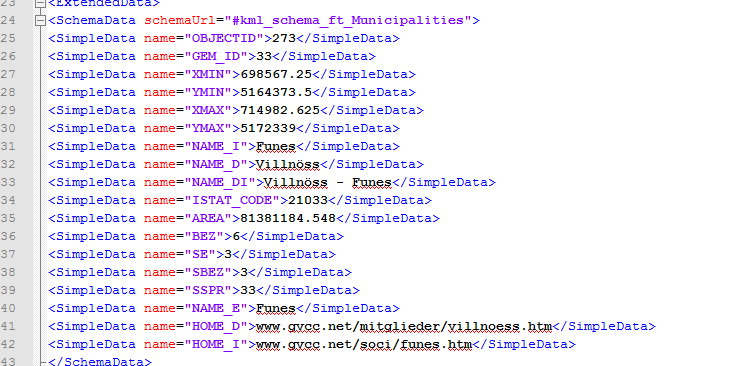# Android Questionpolyline from kml to txt

#### arnold steger

##### Member
Longtime User
I need help for this question.
Want parse a kml file and maked from any Polyline a txt file.
I have a problem when want finde a name for separatly the polyline and convert my string whit all my latlon in a list.
step 1
read file, in <td> is my name (273, second polyline 274) for my filename
step 2
convert <coordinates> in a list and saved as txt-file

I dont finde a way to read the data <td> and <coordinates>
I need Text as Double for write any latlon in a list
in my compled kml file are 300 polyline to convert.

B4X:
``````Sub Globals
Dim TempName,TempAllCoords,TempCoords As String
Dim TempAllList,SafeList As List

End Sub
Sub Parser_EndElement (Uri As String, Name As String, Text As StringBuilder)
If parser.Parents.IndexOf("Document") > -1 Then
If Name="td" Then
TempName=Text.ToString
End If

If Name = "coordinates" Then
SafeList.Initialize
TempAllCoords=Text.ToString
TempCoords=TempAllCoords.Replace("0 ","")
Dim PolygonCoords1 () As Double=Array As Double(TempCoords) 'to convert string to double
Dim PolygonPoints1 As List=CoordsToLatLngList1(PolygonCoords1)
For i = 1 To PolygonPoints1.Size-1
Dim PointsTemp() As String = Regex.Split(",",PolygonPoints1.Get(i))
Dim ln As LatLng
ln.Initialize(PointsTemp(0),PointsTemp(1))
Next

Dim Writer As TextWriter
Writer.Initialize(File.OpenOutput(File.DirRootExternal & "/Grenzen",TempName&".txt",True))
Writer.WriteList(SafeList)
Writer.Close
End If
End If

End Sub

Sub CoordsToLatLngList1(PolygonCoords1() As Double) As List
Dim PolygonCoords1Count As Int=PolygonCoords1.Length
Dim LatLngList1 As List
LatLngList1.Initialize``````

#### Attachments

• KML.txt
14.3 KB · Views: 404
•Milton FS Santos

#### Erel

##### B4X founder
Staff member
Longtime User
Note that you should almost never use TextWriter. Just call File.WriteList if you want to write a list of text items.

Do you want to get the name from one of these fields?#### eurojam

##### Well-Known Member
Longtime User
Arnold,
you can use the xmlsax library, which makes it easier to read kml, which is xml:
B4X:
``````ub Process_Globals
Dim parser As SaxParser
End Sub

Sub Globals
Dim coords As String
End Sub

Sub Activity_Create(FirstTime As Boolean)
If FirstTime Then
parser.Initialize
End If
'parse the xml file
Dim in As InputStream
in = File.OpenInput(File.DirAssets, "kml.txt")
parser.Parse(in, "Parser")
in.Close
End Sub
Sub Parser_EndElement (Uri As String, Name As String, Text As StringBuilder)
'Log(Name)
If parser.Parents.IndexOf("Polygon") > -1 Then
If Name = "coordinates" Then
coords = Text.ToString
Log(coords)
End If
End If
End Sub``````

•Milton FS Santos

#### arnold steger

##### Member
Longtime User
I have tested this code. When build latlon from string show error.
line: LatLng1.Initialize(PolygonCoords1(i), PolygonCoords1(i+1))

** Activity (main) Create, isFirst = true **

Error occurred on line: 89 (main)
java.lang.NumberFormatException: Invalid double: "11.7406864345806,46.665959060293,11.7404485709996,46.6660862601944 and more....
Message longer than Log limit (4000). Message was truncated.

B4X:
``````Sub Parser_EndElement (Uri As String, Name As String, Text As StringBuilder)
If parser.Parents.IndexOf("Document") > -1 Then
If Name="SimpleData" Then
If NameCount=0 Then
TempName=Text.ToString
End If
If NameCount=7 Then
TempName=TempName&"_"&Text.ToString
End If
NameCount=NameCount+1
End If

If Name = "coordinates" Then
SafeList.Initialize
TempAllCoords=Text.ToString
TempCoords=TempAllCoords.Replace("0 ","")
TempAllList.Initialize
TempAllList=Regex.Split(",",TempCoords)

Dim PolygonCoords1 () As String=Array As String(TempCoords)
Dim PolygonPoints1 As List=CoordsToLatLngList1(PolygonCoords1)
For i = 1 To PolygonPoints1.Size-1
Dim PointsTemp() As String = Regex.Split(",",PolygonPoints1.Get(i))
Dim ln As LatLng
ln.Initialize(PointsTemp(0),PointsTemp(1))
Next

File.WriteList(File.DirRootExternal& "/Grenzen",TempName&".txt",SafeList)
NameCount=0
End If
End If

End Sub

Sub CoordsToLatLngList1(PolygonCoords1() As String) As List

Dim LatLngList1 As List
LatLngList1.Initialize
Dim i As Int
For i=0 To TempAllList.Size/2 Step 2
Dim LatLng1 As LatLng
LatLng1.Initialize(PolygonCoords1(i), PolygonCoords1(i+1))
Next
Return LatLngList1
End Sub``````

#### eurojam

##### Well-Known Member
Longtime User
try
B4X:
``````Sub Parser_EndElement (Uri As String, Name As String, Text As StringBuilder)
'Log(Name)
If parser.Parents.IndexOf("Document") > -1 Then
If Name = "coordinates" Then
Dim l, cl As List
cl.Initialize
l.Initialize
coords = Text.ToString
coords = coords.Replace("0 ","")
Log(coords)
l = Regex.Split(",",coords)
Log(l)
For i=0 To l.Size/2 Step 2
Dim ll As LatLng
ll.Initialize(l.Get(i), l.Get(i+1))

Next
Log (cl)
End If
End If

End Sub``````

•arnold steger

#### arnold steger

##### Member
Longtime User
You are the best. Thanks
This code work perfectly.
B4X:
``````Sub Globals
Dim ListView1 As ListView
Dim TempName,TempAllCoords,TempCoords As String
Dim TempAllList,SafeList As List
Dim NameCount As Int
End Sub
Sub Parser_EndElement (Uri As String, Name As String, Text As StringBuilder)
If parser.Parents.IndexOf("Document") > -1 Then
If Name="SimpleData" Then
If NameCount=0 Then
TempName=Text.ToString
End If
If NameCount=7 Then
TempName=TempName&"_"&Text.ToString
End If
NameCount=NameCount+1
End If

If Name = "coordinates" Then
SafeList.Initialize
TempAllCoords=Text.ToString
TempCoords=TempAllCoords.Replace("0 ","")
TempAllList.Initialize
TempAllList=Regex.Split(",",TempCoords)

For i=0 To TempAllList.Size/2 Step 2
Dim ln As LatLng
ln.Initialize(TempAllList.Get(i+1),TempAllList.Get(i))
Next

File.WriteList(File.DirRootExternal& "/Grenzen",TempName&".txt",SafeList)
NameCount=0
End If
End If

End Sub``````